library(ggplot2)
library(ggpattern)

## Introduction pattern = 'crosshatch'

The crosshatch pattern

## Pattern Parameters

aesthetic description default possible values
pattern_colour Stroke colour ‘grey20’ colour
pattern_fill Fill colour of ‘horizontal’ lines ‘grey80’ colour
pattern_fill2 Fill colour of ‘vertical’ lines NA colour
pattern_angle Rotation angle 30 angle in degrees
pattern_density Approx. fraction of area the pattern fills 0.2 value in range [0, 1] (fraction)
pattern_spacing Spacing between repetitions of pattern 0.05 value in range [0, 1] (snpc units)
pattern_xoffset Shift pattern along x axis 0 value in range [0, 1] (snpc units)
pattern_yoffset Shift pattern along y axis 0 value in range [0, 1] (snpc units)
pattern_alpha Alpha NA value in range [0, 1] or NA
pattern_linetype Stroke linetype 1 linetype
pattern_size Stroke linewidth 1 linewidth

There are also a number of parameters for extra control of legend sizing and aspect ratio adjustments. See the ‘Pattern Parameters - Common’ for more information.

## Data

Standard data for all the example plots

df <- data.frame(trt = c("a", "b", "c"), outcome = c(2.3, 1.9, 3.2))
df
#>   trt outcome
#> 1   a     2.3
#> 2   b     1.9
#> 3   c     3.2

## Vanilla ggplot2

ggplot(df, aes(trt, outcome)) +
geom_col(aes(fill=trt),colour='black') +
theme_bw() +
labs(title = "Plain ggplot2")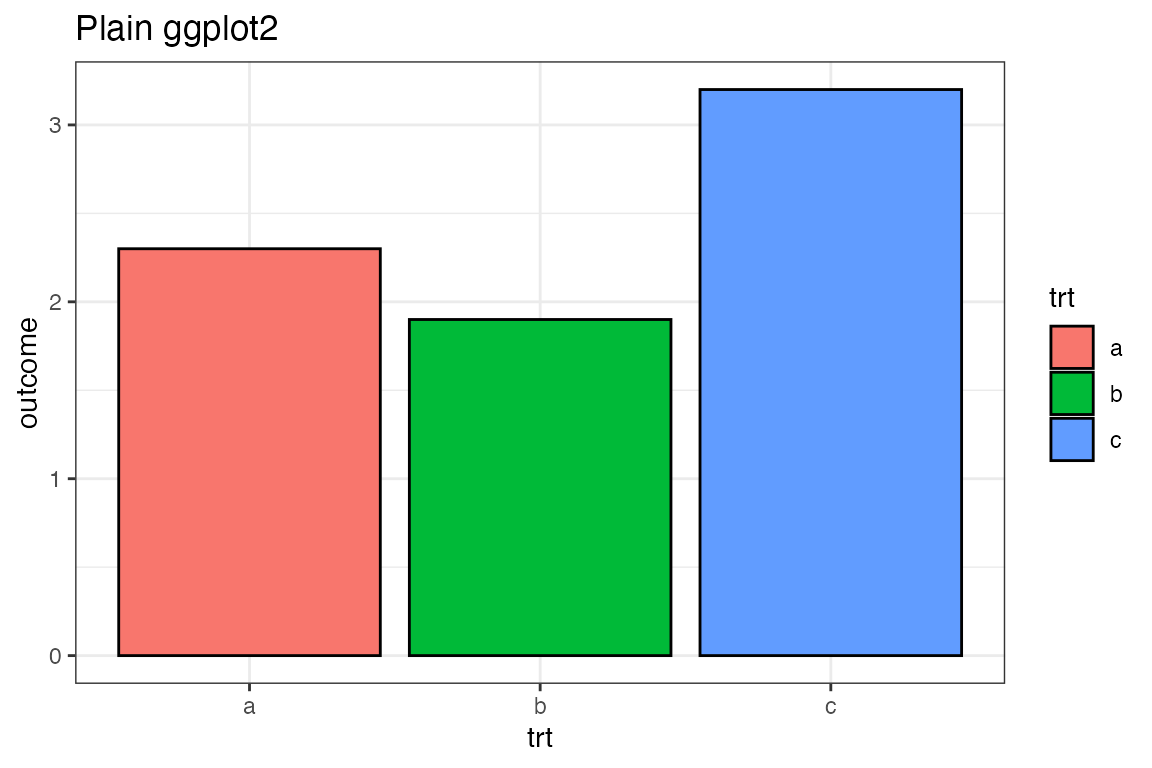## Use the {ggpattern} geom

• Use ggpattern::geom_col_pattern() instead of ggplot2::geom_col().
• Set pattern = 'crosshatch'
ggplot(df, aes(trt, outcome)) +
geom_col_pattern(aes(fill=trt),colour='black', pattern = 'crosshatch') +
theme_bw() +
labs(title = "ggpattern")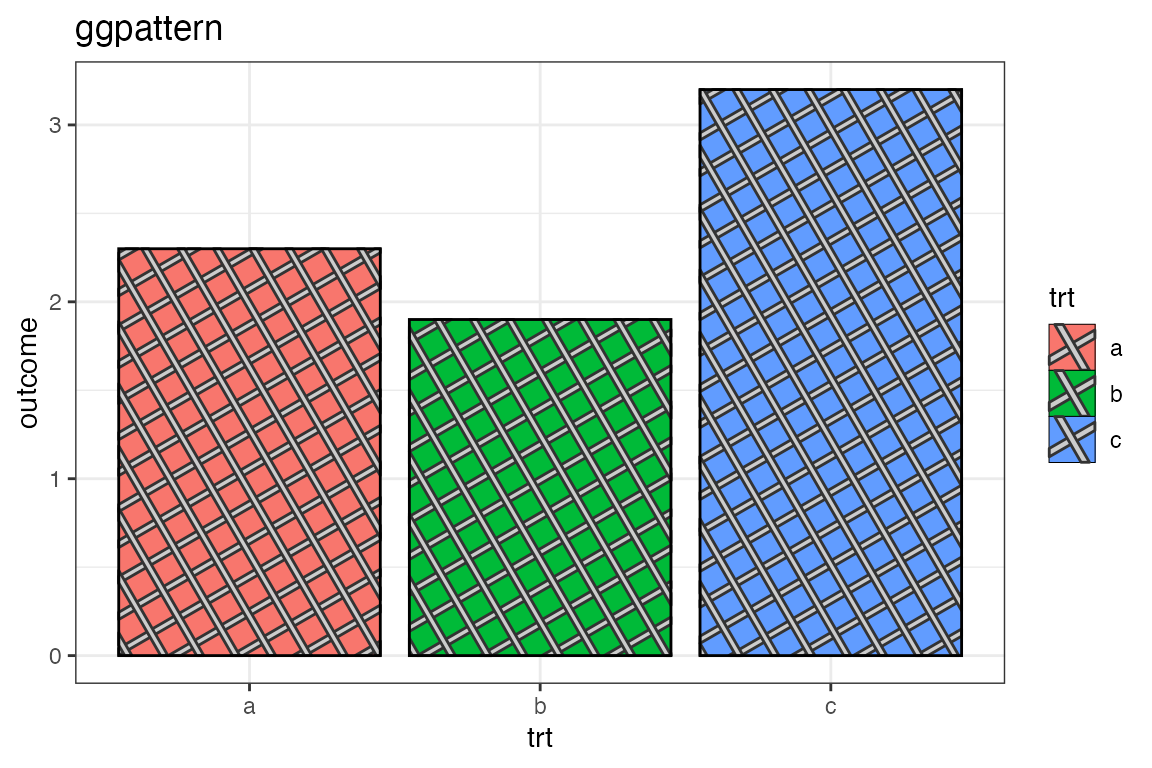## Use coord_fixed()

Due to the underlying nature of grid graphics and how ggplot2 sets up a plot, it is essential to use coord_fixed() in order to achieve consistent looking patterns. Without a fixed coordinate system, then ggpattern has to make guesses on some sizes, and patterns may look askew e.g. 90 degree angles in patterns may no longer appear properly!

ggplot(df, aes(trt, outcome)) +
geom_col_pattern(aes(fill=trt),colour='black', pattern = 'crosshatch') +
theme_bw() +
labs(title = "ggpattern + coord_fixed()") +
coord_fixed(ratio = 1/2)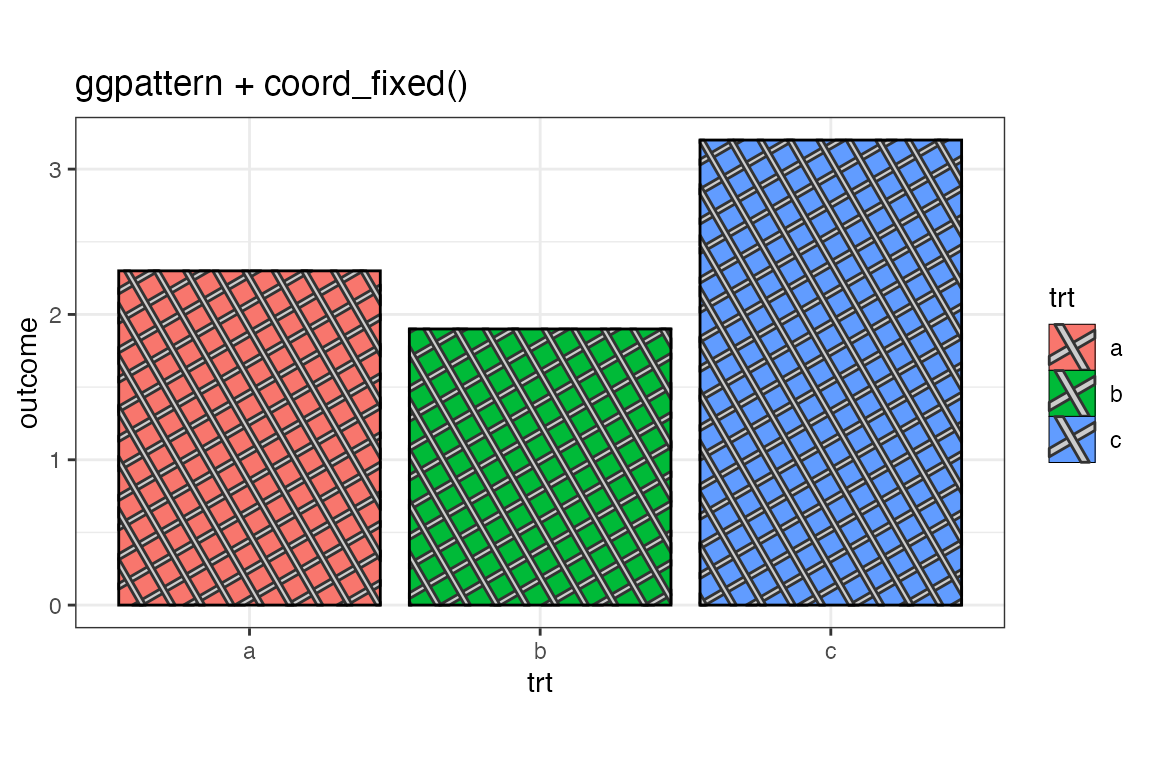## Bigger Legend Keys

Default legends in ggplot2 are usually too small to show off an example of the pattern, so it will usually be necessary to increase the key size.

ggplot(df, aes(trt, outcome)) +
geom_col_pattern(aes(fill=trt),colour='black', pattern = 'crosshatch') +
theme_bw() +
labs(title = "ggpattern + coord_fixed()") +
coord_fixed(ratio = 1/2) +
theme(legend.key.size = unit(1.5, 'cm'))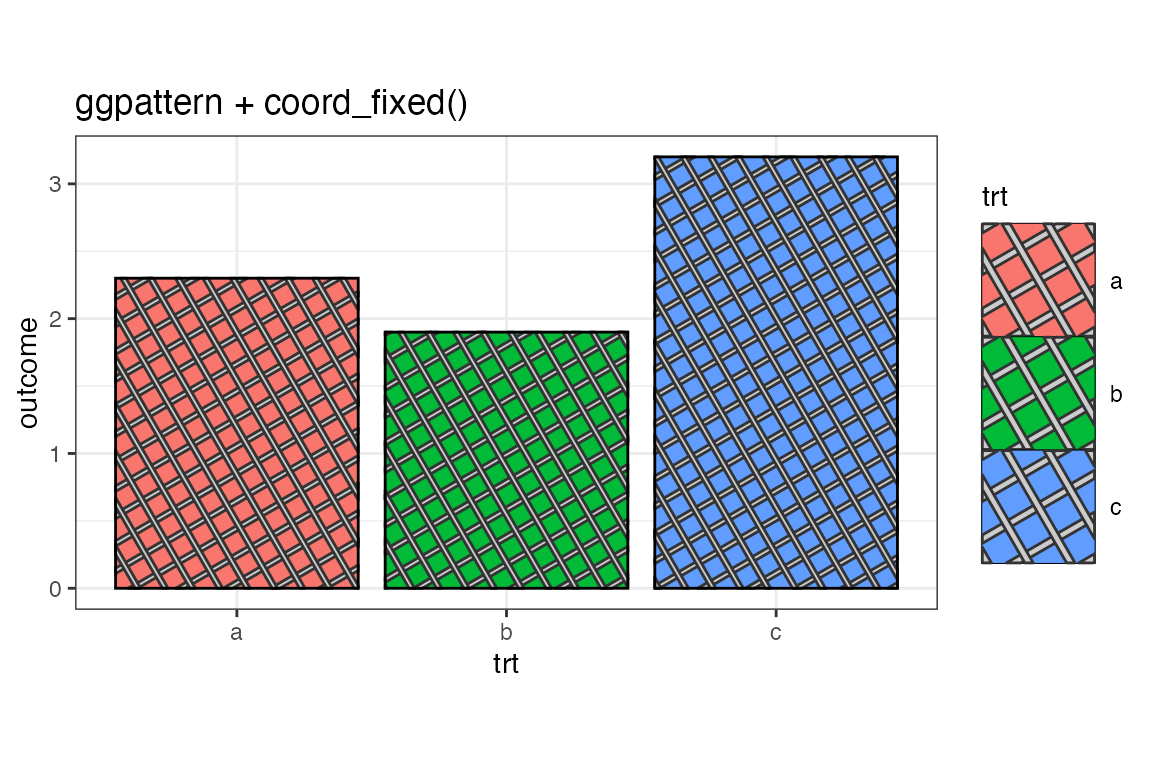## The Density Aesthetic

The aesthetic pattern_density roughly corresponds to the fraction of the filled area which should be covered by the pattern.

In the following plot the density of striping is increased to 50% of the fill area.

ggplot(df, aes(trt, outcome)) +
geom_col_pattern(
aes(fill=trt),
colour          = 'black',
pattern         = 'crosshatch',
pattern_density = 0.5
) +
theme_bw() +
labs(title = "Fixed density of 0.5 (50% of the fill area)") +
coord_fixed(ratio = 1/2) +
theme(legend.key.size = unit(1.5, 'cm'))## The Density Aesthetic as a Mapped Aesthetic

ggplot(df, aes(trt, outcome)) +
geom_col_pattern(
aes(fill = trt, pattern_density = trt),
colour          = 'black',
pattern         = 'crosshatch'
) +
theme_bw() +
labs(title = "Aesthetic Mapping of 'trt' to Density") +
coord_fixed(ratio = 1/2) +
theme(legend.key.size = unit(1.5, 'cm'))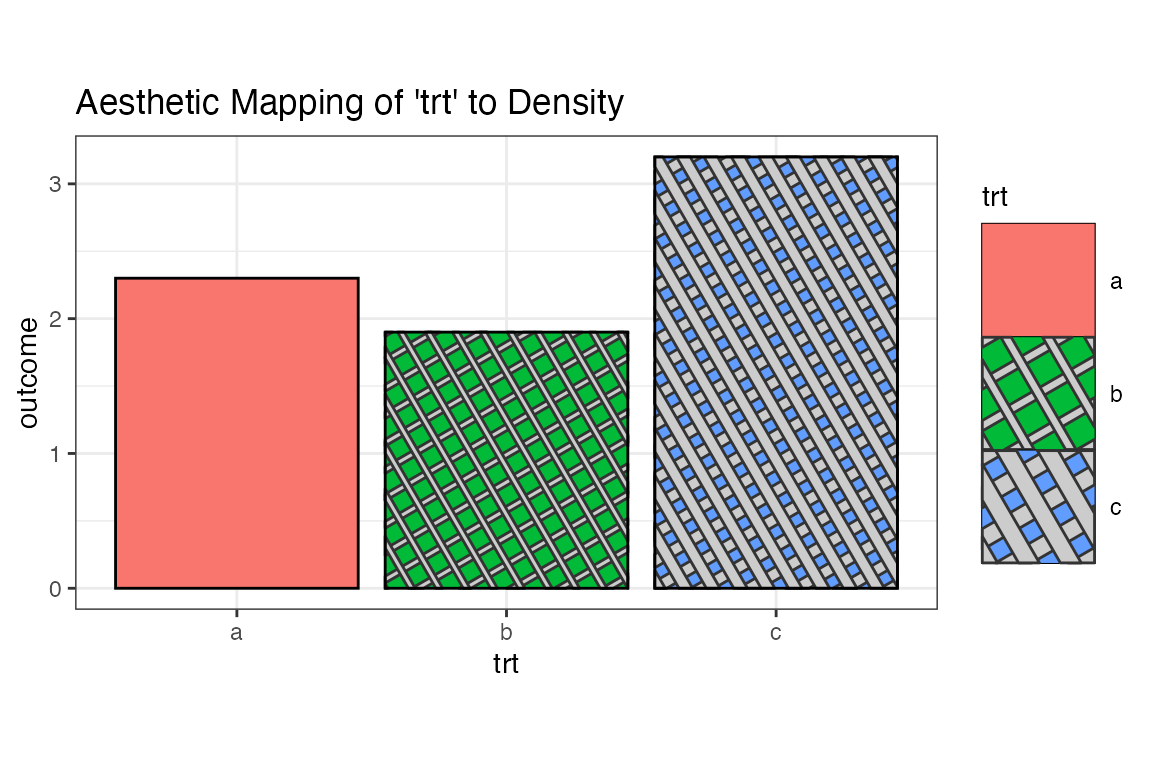## The Density Aesthetic as a Mapped Aesthetic with Manual Scale

scale_pattern_density_manual() can be used to manually control how the variable is mapped to the density.

ggplot(df, aes(trt, outcome)) +
geom_col_pattern(
aes(fill = trt, pattern_density = trt),
colour          = 'black',
pattern         = 'crosshatch'
) +
theme_bw() +
labs(title = "Aesthetic Mapping of 'trt' to Density") +
coord_fixed(ratio = 1/2) +
theme(legend.key.size = unit(1.5, 'cm')) +
scale_pattern_density_manual(values = c(a = 0.1, b=0.3, c=0.5))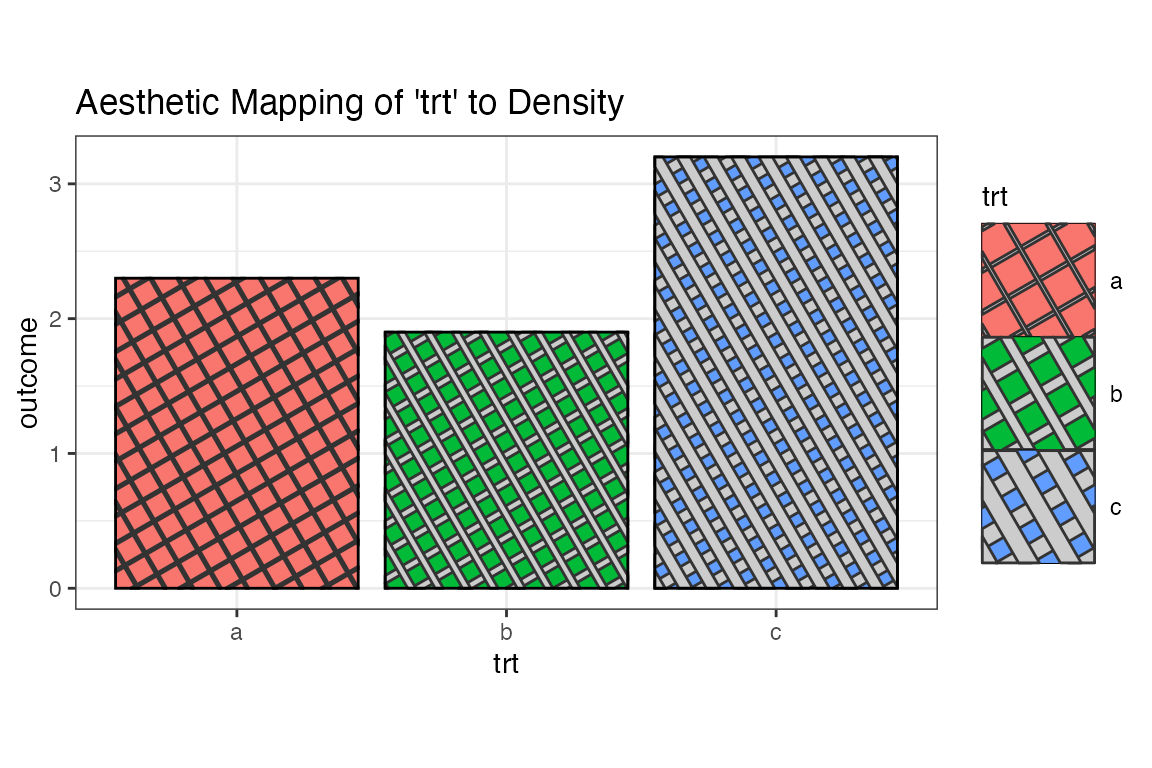## The Spacing Aesthetic as a Mapped Aesthetic

ggplot(df, aes(trt, outcome)) +
geom_col_pattern(
aes(fill = trt, pattern_spacing = trt),
colour          = 'black',
pattern         = 'crosshatch'
) +
theme_bw() +
labs(title = "Aesthetic Mapping of 'trt' to Spacing") +
coord_fixed(ratio = 1/2) +
theme(legend.key.size = unit(1.5, 'cm'))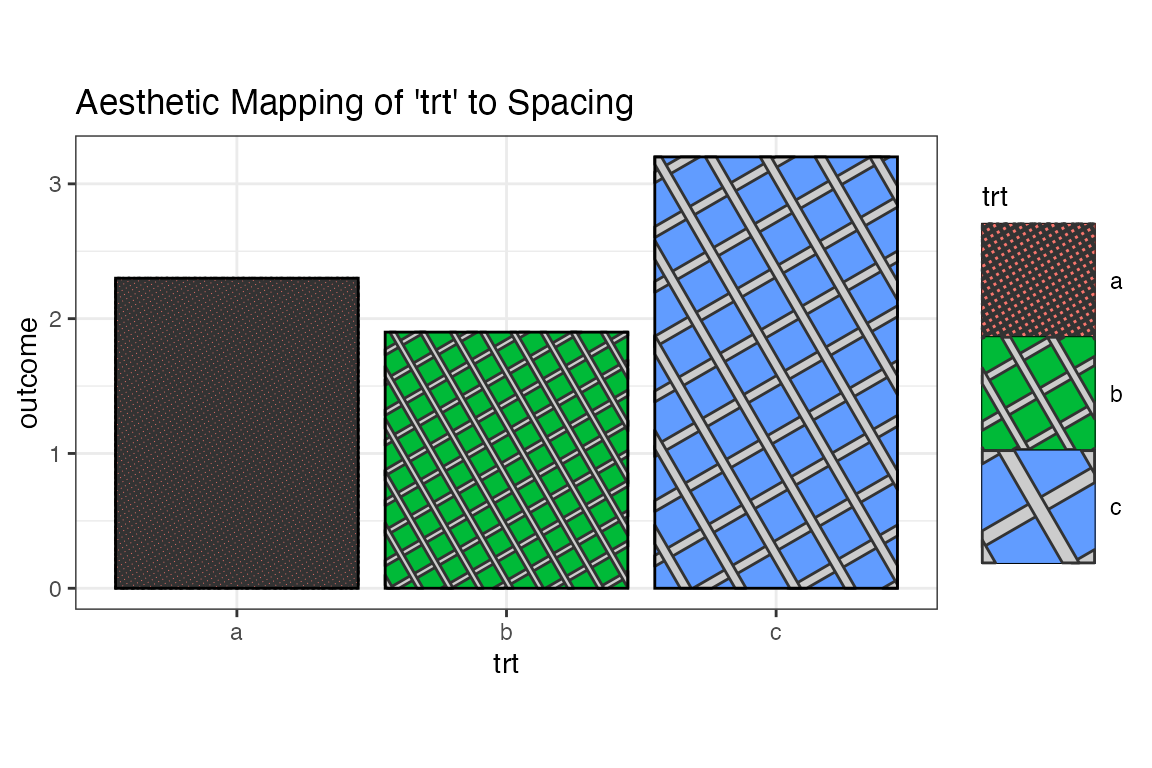## The Fill Aesthetic as a Mapped Aesthetic

ggplot(df, aes(trt, outcome)) +
geom_col_pattern(
aes(fill = trt, pattern_fill = trt),
colour          = 'black',
pattern         = 'crosshatch'
) +
theme_bw() +
labs(title = "Aesthetic Mapping of 'trt' to Pattern Fill") +
coord_fixed(ratio = 1/2) +
scale_pattern_fill_viridis_d() +
theme(legend.key.size = unit(1.5, 'cm'))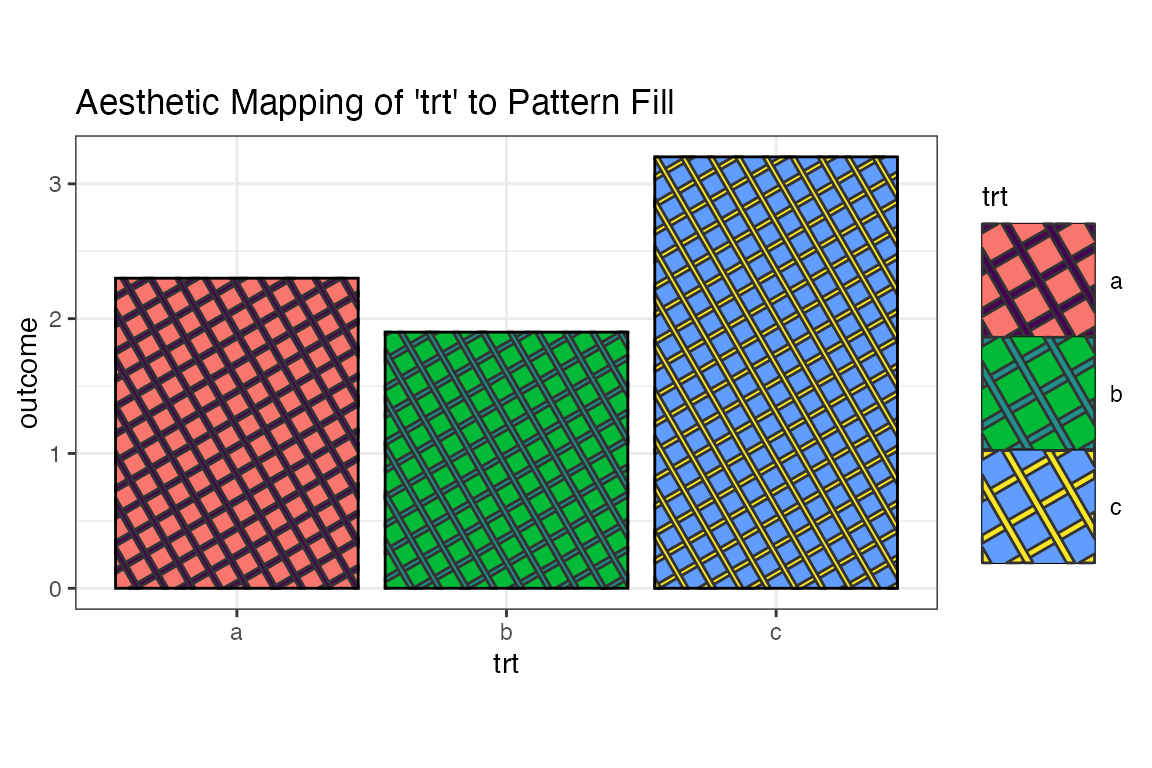## Technical Bits

• Crosshatches are implemented as two overlapping stripe patterns.
• The clipping of the crosshatch polygons is done by creating the crosshatches as sf objects, performing an sf::st_intersection() with the element boundary, and then converting the clipped crosshatches back into a grid::polygonGrob().
• If lines had been used for the crosshatches, then there would be visual artefacts where the crosshatch intersects the boundary because the lineend graphic parameter can only be square, round etc, and cannot conform to the actual shape of the element boundary.